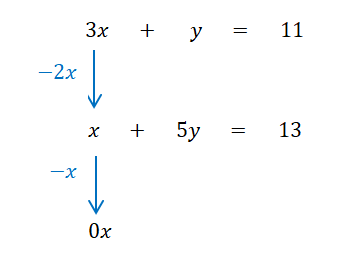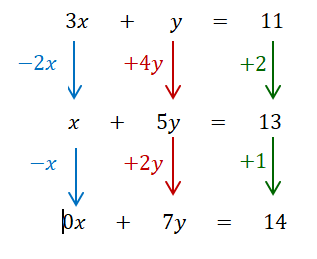Thursday, 31 May 2018

Simultaneous equations: an insight from playing with technology

I've noticed a couple of tweets recently about the importance of being playful when doing mathematics and this has brought to mind an insight I had about simultaneous linear equations that occurred when I was being playful with them in technology. As a result of this I now have a different method for solving that I  prefer to the standard textbook approaches.

Playing with simultaneous equations

In trying to construct a pair of linear simultaneous equations where the solution went through a given point. I can't remember the point but I'll use (3,2) for the example. I did this by creating a point A at (3,2) then two more points B and C and finding the lines that went through these and A. This gave me the simultaneous equations:
3x + y = 11
x + 5y = 13

I then moved the points B and C around to find some different equations that would have the same point of intersection. This is probably best displayed here by showing all the different lines I got in a different colour.

The equations for these lines are:
4xy = 10
3x + y = 11
2x + 3y = 12
x + 5y = 13
y = 2

By playing around with these I noticed some interesting features:
• There are infinitely many lines through the point A.
• The coefficients of these lines are related linearly.
• One of these lines will be horizontal and one will be vertical (and hence have a simple equation just in terms of x or y).
NB rewriting the final equation as 0x + 7y = 14 makes the linear relationship more obvious.

A simple method for solving linear simultaneous equations

Putting all this together I realised this could be used to form a simple method for solving simultaneous linear equations. They can be solved by moving linearly along the coefficients until one of the coefficients of either x or y is 0. For example:

3x + y = 11
x + 5y = 13

The gap from 3x to x is –2x, so if you move half this gap again (–x) from x you'll have 0x.Moving an equivalent amount for the other terms means you need to move half the gap from y to 5y, i.e. +2y, on from 5y to get 7y and half the gap from 11 to 13, i.e. +1, to get 14.This gives 0x + 7y = 14 and hence y = 2. Then using the first equation 3x = 9 gives x = 3.

I've tried this method with lots of linear simultaneous equations and I think it's quicker and makes more sense to me. It's the method I know use if I need to solve them.

The importance of being playful

This method only occurred to me because I was being playful with the mathematics in the technology. The dynamic aspect of technology offers a fantastic opportunity to be playful with mathematics as it allows the users to change aspects of a situation and observe the impact on representations (here it was graphical and algebraic but it applies to others such as geometrical or numerical too). The playfulness here is not in the sense of 'lets have fun messing around' but being open to explore a situation and then try to explain what is happening and why.

1 comment:

1.Very nice blogs!!! I have learned a lot of information for this sites...thanks for Sharing wonderful blog.educational software